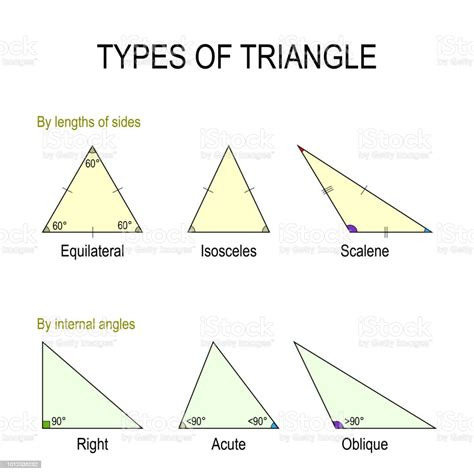# Generate Triangular Circle w/ Function

Original Post

I’m trying to generate a circle using equilateral triangles, but I can’t seem to figure out how to calculate the rotation and amount of needed triangles, based on the width (which I’m currently testing with just an even equilateral triangle for simplicity’s sake).

I also want to calculate this based on the length of the triangle when it’s longer (which I assume is just the radius).

2 Likes

Sorry I don’t see how you’d make a circle out of equilateral triangles. Are you sure you don’t mean right triangles?If not, could you draw an example of what you mean? The image you posted doesn’t help

Well, I’m putting two right triangles together to make an equilateral triangle. I guess the even length can’t properly form a circle. I’m essentially just doing trial and error from the very start, so I don’t really know that length each triangle part should be.

But I’m basically trying to form a complete circle with triangle parts.

1 Like

Oh! Well, yeah you can form any triangle from two right triangles. With a bunch of isosceles triangles you could form a circle iteratively. Start with an equilateral, then you can subdivide a side into two smaller sides like this:

Is this what you’re going for?

Ah sorry, that’s not quite what I meant. But here’s an image I found that’s a better example of what I meant:

1 Like

It’s impossible to make equilateral triangles of a circle as circle sectors. The sum of degrees should be 180, but equilateral triangles require the third side to also be equal in length(which can’t, it’s a segment of about a half circle). If you meant something like pizza slices(sectors), you need to find the radius and degrees per sector.

Those are Isosceles triangles isnt? a circle shape could be built using many of them taking in count the size of the smaller side I think

If its an equilateral triangle you need exactly 6 and the angle between each is 60 degrees.

This is what happens when I use an equilateral triangle with 60 degrees:

How exactly would I do that?

Those aren’t equilateral triangles. How did you make those?

These aren’t even triangles. Look at the shortest edge. This is impossible. I see really no way to do this other than using multiple meshes, or A LOT of small triangles.

These are two wedges with half width put together. The total length of the triangle is 1 stud all around, so all corners should be completely equal.

Could you explain why you need to do it this way? So we could offer better methods if possible?

The most accurate you’re going to get with equilateral triangles is with a hexagon. The only way you’ll get anymore accurate is by utilizing isoceles.

I’m attempting to make a circular radius that can be blocked by obstacles. My plan was to shoot rays in every direction and make the triangles length based on how far the ray actually got, then fill in the empty space with red.

Could you send an example, please?

I don’t really have an example because I don’t have anything that’s working. I just want to know how to make a complete circle with triangles right now, as shown here:

I’m thinking of using a triangle mesh to avoid having to calculate the triangle itself, but I still need to know how to calculate the size and rotation required to make a complete circle. I’d also like to be able to have it be calculated based on the number of triangles I want to use, which would be determined by the actual size of the circle that I want.

I should clarify that I’m obviously not looking for round edges as no circle can truly be round. I’m just going to use more triangles to “smooth” out the edge to make it appear round.

1 Like

The problem with this method is that those aren’t triangles. They’re eighths of a circle. So yes, you should probably use a mesh, or a lot of isoceles triangles.

I’m not certain what you mean, but this is what the math should look like if you’re using two right triangles.

Those are essentially triangles. But anyway, those are just semantics. I’m still trying to figure out the math needed to make it work.